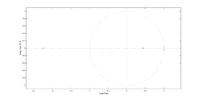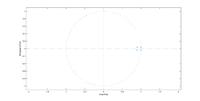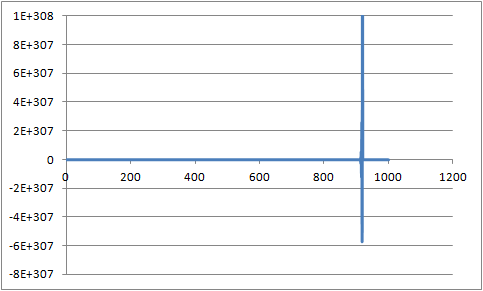# Digital iir filter stability checking.

1. ## Digital iir filter stability checking.

Hi all,

I am trying to confirm the stability of some digital iir filters I am using.

I have downloaded a trial of Matlab 2014b and have been attempting to use the isstable function to check stability, this tells me that my high frequency notch and bandpass filters are stable but my mains (50Hz) noise notch filter and my 10Hz high pass filter are not.

they both show one pole at around -2.4 + 0i as shown in the image below.this is obviously why isstable is returning 0, so I tried designing a notch filter with both poles and zeros at +/- 2.25 degrees (8KHz sample rate) with a radius of 1 for zeroes and 0.9 for the poles. as can be seen in the picture below.this generated a decent looking frequency response on matlab using the freqz function but transferring to a practical application of the filter in excel produced an output which oscillated out of control when subject to an impulse (value of 1) at sample #5 and all remaining samples where 0.my a and b coefficients were generated from matlab using the poly(n) function where n = zero locations to generate my b coefficients and n = pole locations to generate my a coefficients.

this gave me
a = [1, -1.7986122652, 0.809999999964175]
b = [1, -1.9984580724, 0.999999999913979]

the above excel graph was generated using the formula:

=SUM((\$C\$2*B9)+(\$D\$2*B8)+(\$E\$2*B7)+(\$D\$3*C8)+(\$E\$3 *C7))

where D3,E3 are my b coefficients, C2,D2,E2 are my a coefficients, B9-7 are my current and two previous input samples and C8-7 are my two previously filtered outputs.

I do not understand this, Matlab says the coefficients should provide a stable filter but excel is saying otherwise!!! can anyone see an issue with my workings of anything that I may have overlooked.

Any help would be much appreciated.•

2. ## Re: Digital iir filter stability checking.

this turned out to be the formula I was using, matlab seems to use the formula equivalent to

=SUM((\$C\$2*B9)+(\$D\$2*B8)+(\$E\$2*B7)-(\$D\$3*C8)-(\$E\$3 *C7))

therefore inverting my b coefficients fixed the problem.--[[ ]]--